Скачать презентацию Externalities Allen C Goodman 2009 Ideal

f52fa86b7cfc5aa8f744fbbf0f01be17.ppt

• Количество слайдов: 32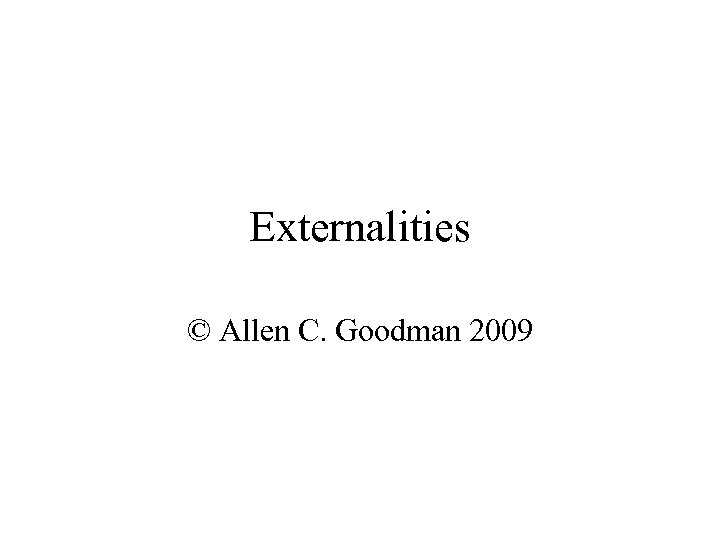Externalities © Allen C. Goodman 2009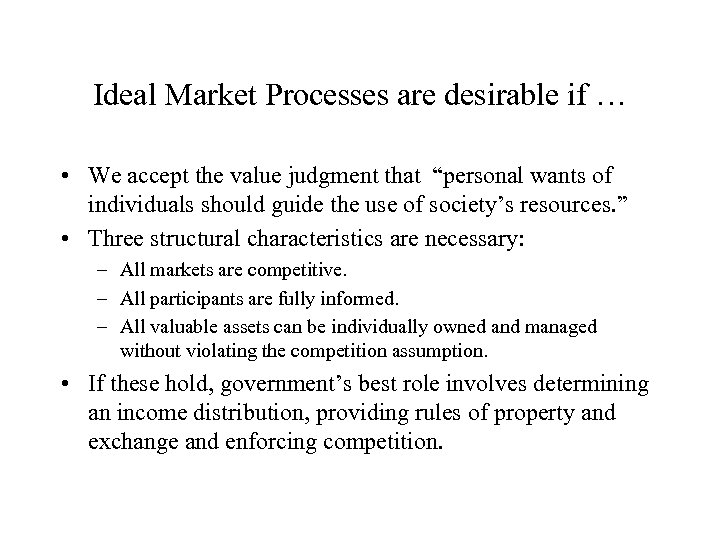Ideal Market Processes are desirable if … • We accept the value judgment that “personal wants of individuals should guide the use of society’s resources. ” • Three structural characteristics are necessary: – All markets are competitive. – All participants are fully informed. – All valuable assets can be individually owned and managed without violating the competition assumption. • If these hold, government’s best role involves determining an income distribution, providing rules of property and exchange and enforcing competition.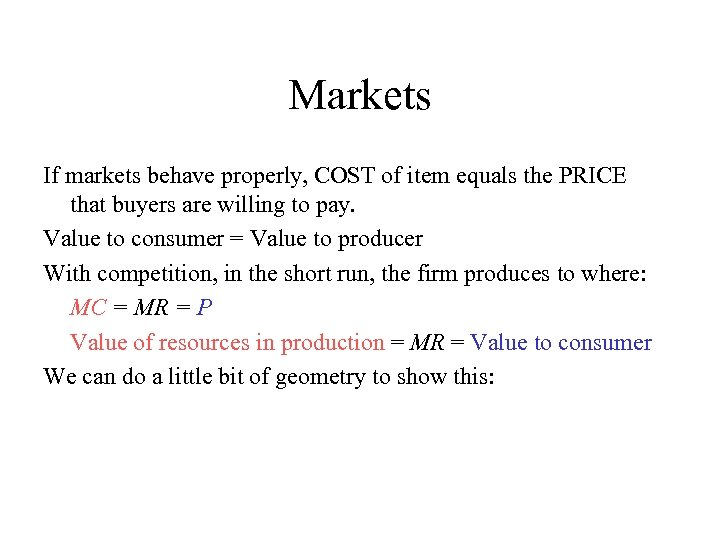Markets If markets behave properly, COST of item equals the PRICE that buyers are willing to pay. Value to consumer = Value to producer With competition, in the short run, the firm produces to where: MC = MR = P Value of resources in production = MR = Value to consumer We can do a little bit of geometry to show this: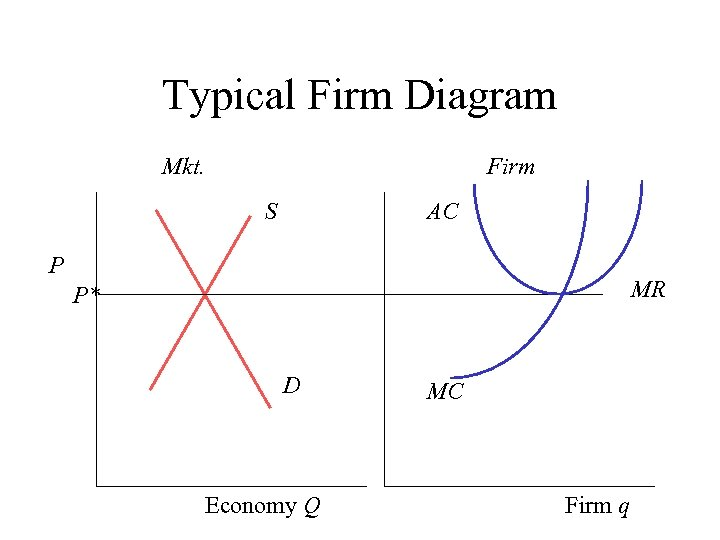Typical Firm Diagram Mkt. Firm S AC P MR P* D Economy Q MC Firm q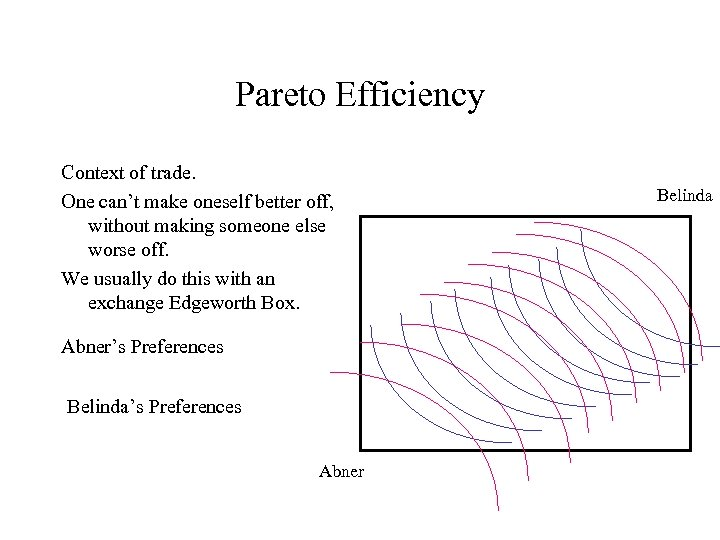Pareto Efficiency Context of trade. One can’t make oneself better off, without making someone else worse off. We usually do this with an exchange Edgeworth Box. Abner’s Preferences Belinda’s Preferences Abner BelindaPareto Efficiency Start at Point A. Is this an Equilibrium? Belinda No, they can trade Belinda can be better off. B So can Abner. A Abner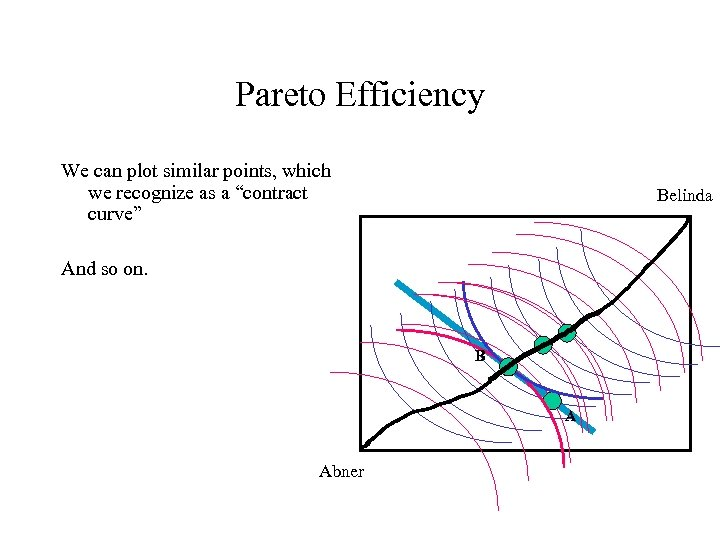Pareto Efficiency We can plot similar points, which we recognize as a “contract curve” Belinda And so on. B A Abner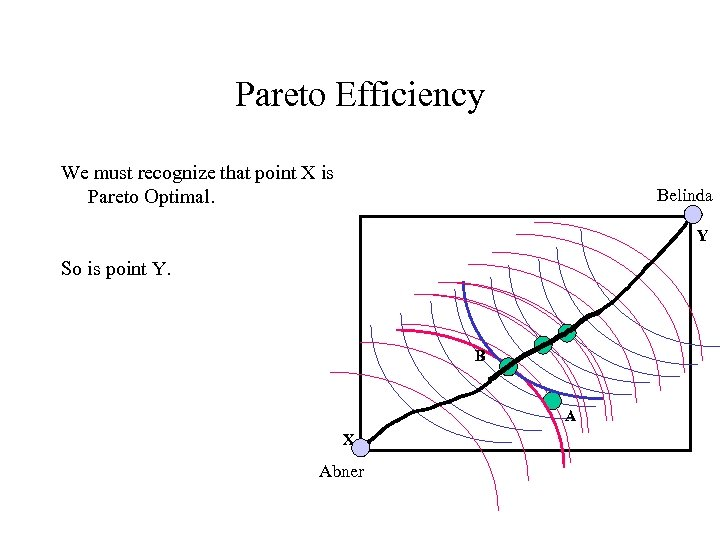Pareto Efficiency We must recognize that point X is Pareto Optimal. Belinda Y So is point Y. B A X Abner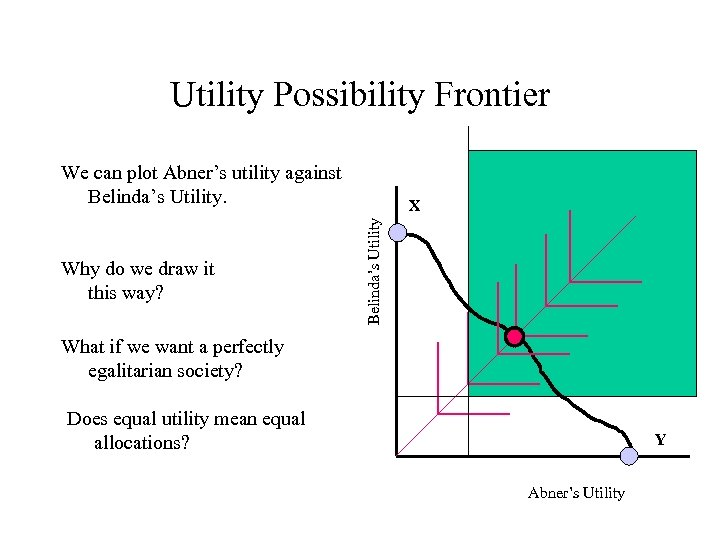Utility Possibility Frontier We can plot Abner’s utility against Belinda’s Utility Why do we draw it this way? X What if we want a perfectly egalitarian society? Does equal utility mean equal allocations? Y Abner’s Utility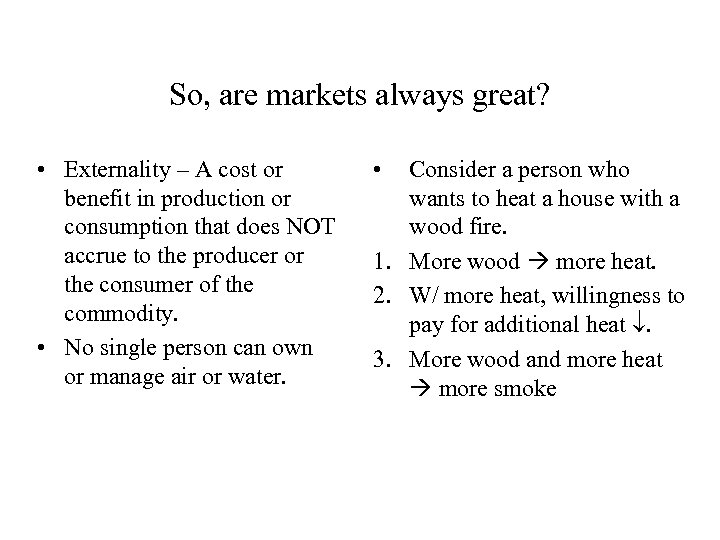So, are markets always great? • Externality – A cost or benefit in production or consumption that does NOT accrue to the producer or the consumer of the commodity. • No single person can own or manage air or water. • Consider a person who wants to heat a house with a wood fire. 1. More wood more heat. 2. W/ more heat, willingness to pay for additional heat . 3. More wood and more heat more smoke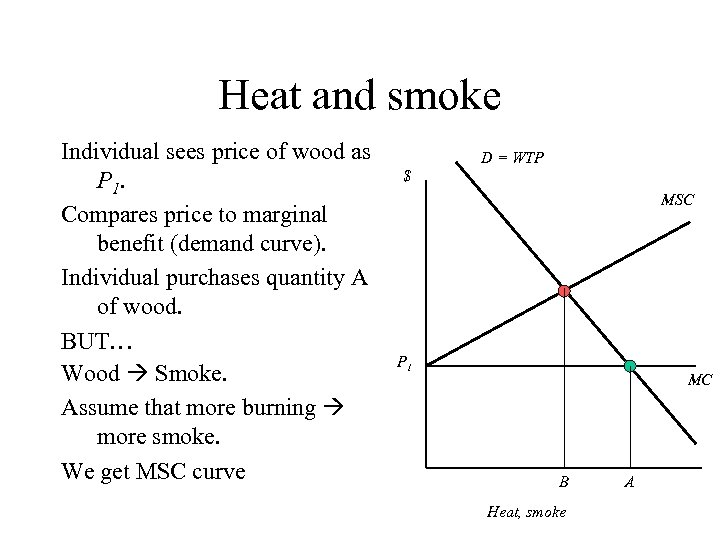Heat and smoke Individual sees price of wood as P 1. Compares price to marginal benefit (demand curve). Individual purchases quantity A of wood. BUT… Wood Smoke. Assume that more burning more smoke. We get MSC curve D = WTP \$ MSC P 1 MC B Heat, smoke A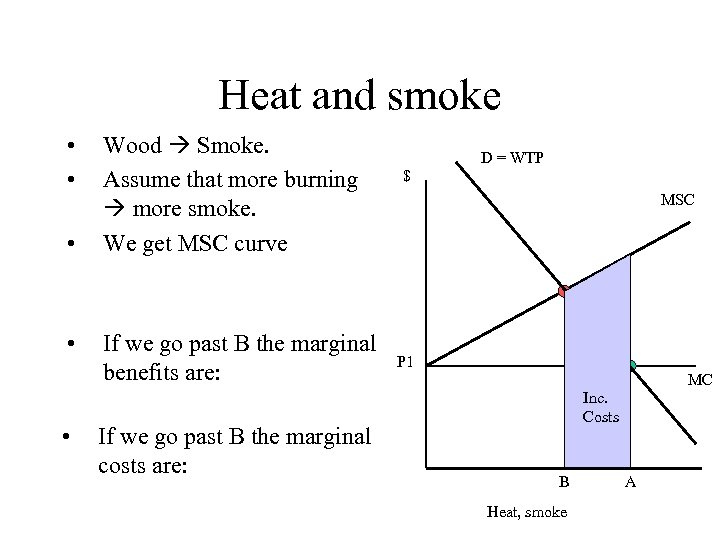Heat and smoke • • • Wood Smoke. Assume that more burning more smoke. We get MSC curve If we go past B the marginal benefits are: If we go past B the marginal costs are: D = WTP \$ MSC P 1 MC Inc. Ben. Costs B Heat, smoke A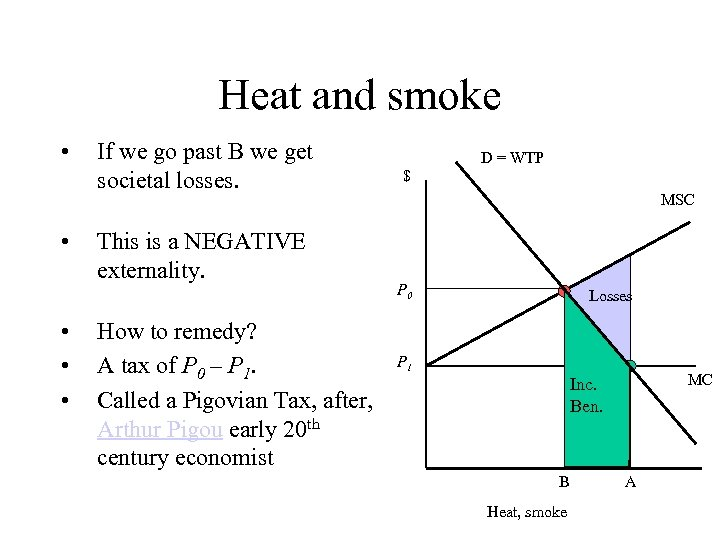Heat and smoke • • • If we go past B we get societal losses. This is a NEGATIVE externality. How to remedy? A tax of P 0 – P 1. Called a Pigovian Tax, after, Arthur Pigou early 20 th century economist D = WTP \$ MSC P 0 Losses P 1 MC Inc. Ben. B Heat, smoke A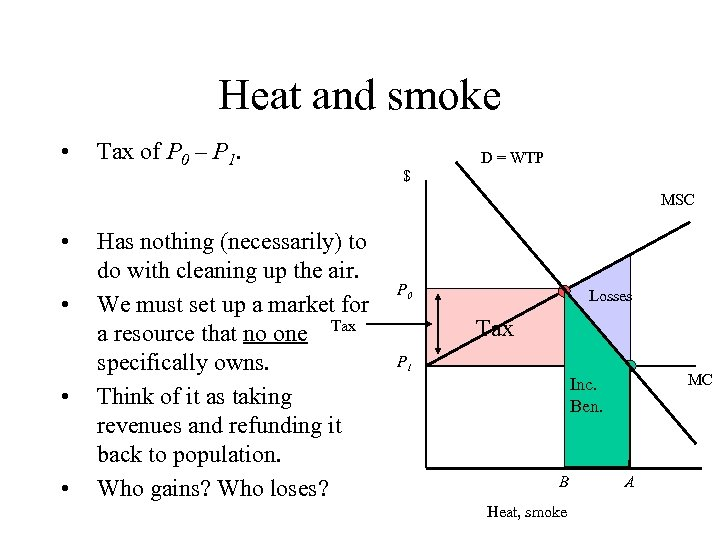Heat and smoke • Tax of P 0 – P 1. D = WTP \$ MSC • • Has nothing (necessarily) to do with cleaning up the air. We must set up a market for a resource that no one Tax specifically owns. Think of it as taking revenues and refunding it back to population. Who gains? Who loses? P 0 Losses Tax P 1 MC Inc. Ben. B Heat, smoke A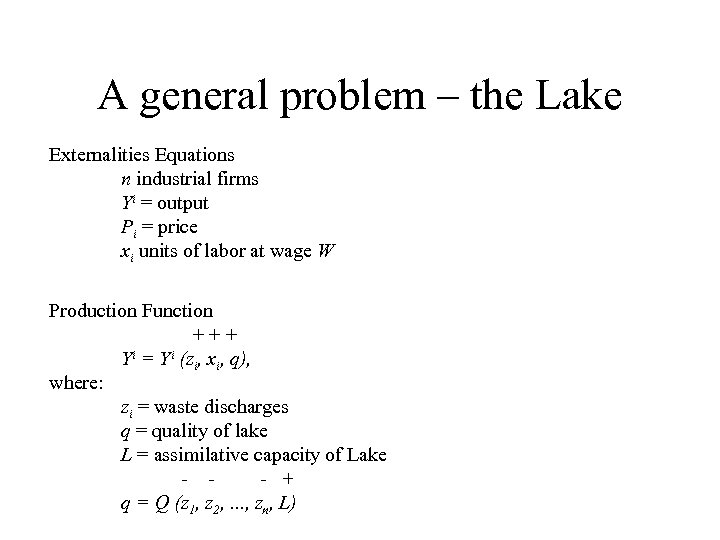A general problem – the Lake Externalities Equations n industrial firms Yi = output Pi = price xi units of labor at wage W Production Function +++ Yi = Yi (zi, xi, q), where: zi = waste discharges q = quality of lake L = assimilative capacity of Lake - - + q = Q (z 1, z 2, . . . , zn, L)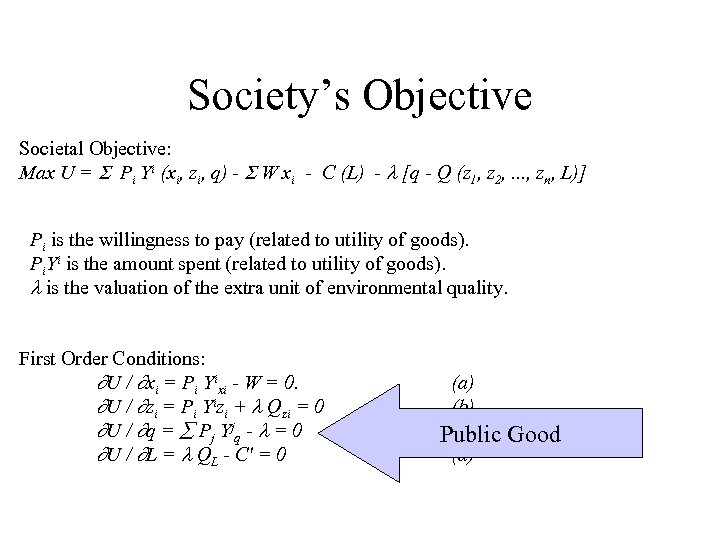Society’s Objective Societal Objective: Max U = Pi Yi (xi, zi, q) - W xi - C (L) - [q - Q (z 1, z 2, . . . , zn, L)] Pi is the willingness to pay (related to utility of goods). Pi. Yi is the amount spent (related to utility of goods). is the valuation of the extra unit of environmental quality. First Order Conditions: U / xi = Pi Yixi - W = 0. U / zi = Pi Yizi + Qzi = 0 U / q = Pj Yjq - = 0 U / L = QL - C' = 0 (a) (b) (c) Public (d) Good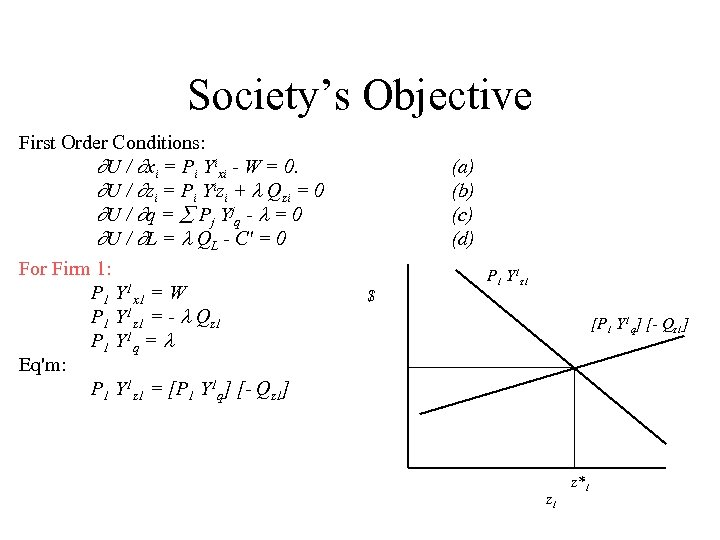Society’s Objective First Order Conditions: U / xi = Pi Yixi - W = 0. U / zi = Pi Yizi + Qzi = 0 U / q = Pj Yjq - = 0 U / L = QL - C' = 0 For Firm 1: P 1 Y 1 x 1 = W P 1 Y 1 z 1 = - Qz 1 P 1 Y 1 q = Eq'm: P 1 Y 1 z 1 = [P 1 Y 1 q] [- Qz 1] (a) (b) (c) (d) \$ P 1 Y 1 z 1 [P 1 Y 1 q] [- Qz 1] z 1 z*1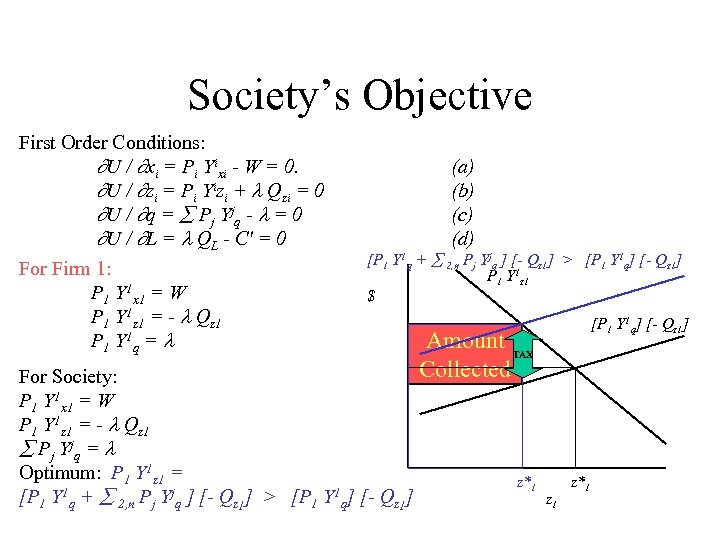Society’s Objective First Order Conditions: U / xi = Pi Yixi - W = 0. U / zi = Pi Yizi + Qzi = 0 U / q = Pj Yjq - = 0 U / L = QL - C' = 0 For Firm 1: P 1 Y 1 x 1 = W P 1 Y 1 z 1 = - Qz 1 P 1 Y 1 q = (a) (b) (c) (d) [P 1 Y 1 q + 2, n Pj Yjq ] [- Qz 1] > [P 1 Y 1 q] [- Qz 1] P 1 Y 1 z 1 \$ For Society: P 1 Y 1 x 1 = W P 1 Y 1 z 1 = - Qz 1 Pj Yjq = Optimum: P 1 Y 1 z 1 = [P 1 Y 1 q + 2, n Pj Yjq ] [- Qz 1] > [P 1 Y 1 q] [- Qz 1] Amount TAX Collected z*1 z 1 z*1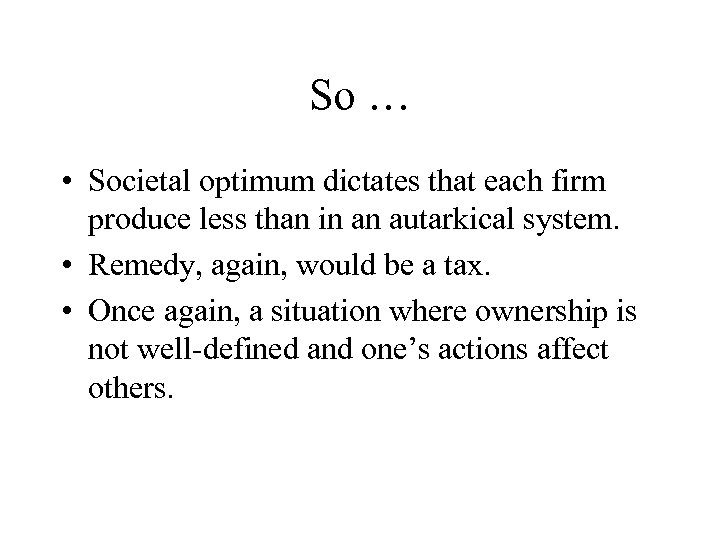So … • Societal optimum dictates that each firm produce less than in an autarkical system. • Remedy, again, would be a tax. • Once again, a situation where ownership is not well-defined and one’s actions affect others.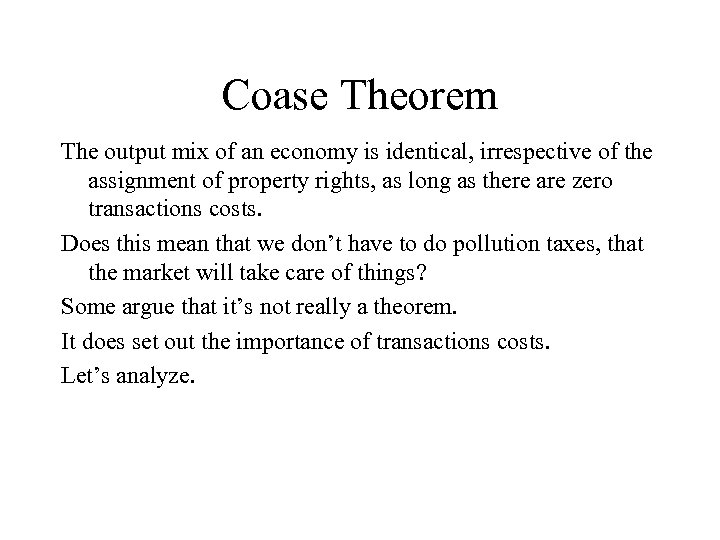Coase Theorem The output mix of an economy is identical, irrespective of the assignment of property rights, as long as there are zero transactions costs. Does this mean that we don’t have to do pollution taxes, that the market will take care of things? Some argue that it’s not really a theorem. It does set out the importance of transactions costs. Let’s analyze.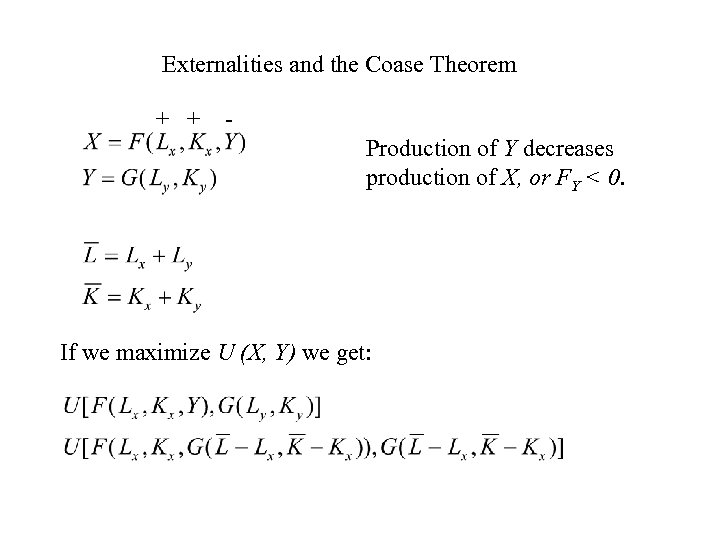Externalities and the Coase Theorem + + Production of Y decreases production of X, or FY < 0. If we maximize U (X, Y) we get: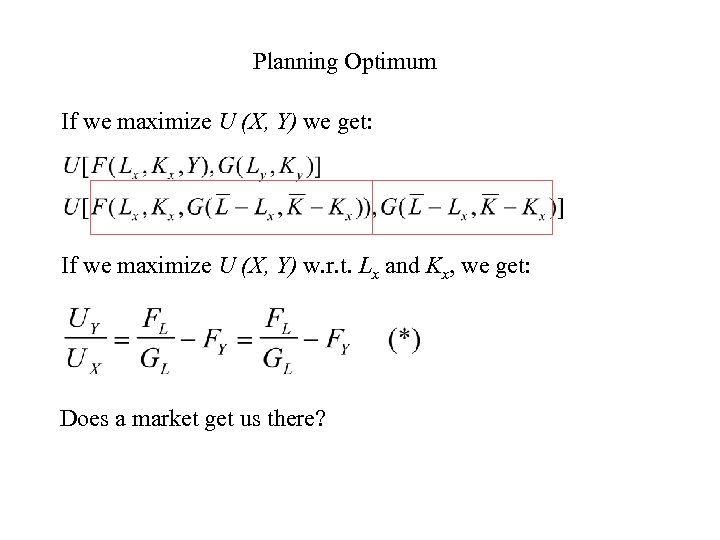Planning Optimum If we maximize U (X, Y) we get: If we maximize U (X, Y) w. r. t. Lx and Kx, we get: Does a market get us there?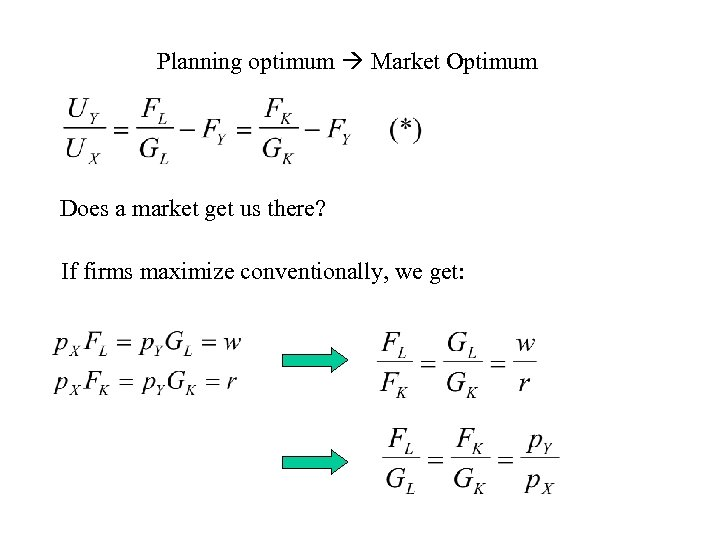Planning optimum Market Optimum Does a market get us there? If firms maximize conventionally, we get: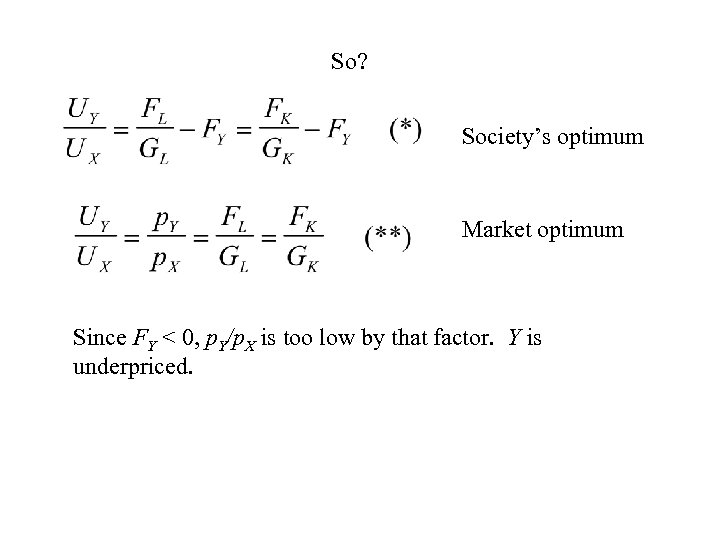So? Society’s optimum Market optimum Since FY < 0, p. Y/p. X is too low by that factor. Y is underpriced.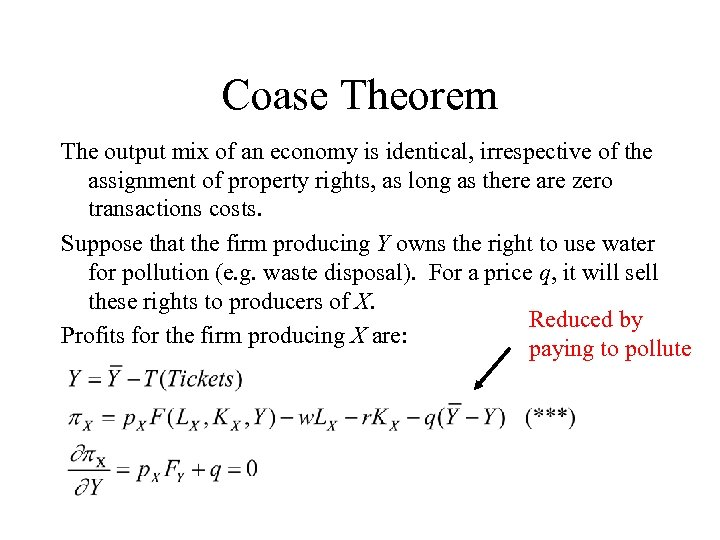Coase Theorem The output mix of an economy is identical, irrespective of the assignment of property rights, as long as there are zero transactions costs. Suppose that the firm producing Y owns the right to use water for pollution (e. g. waste disposal). For a price q, it will sell these rights to producers of X. Reduced by Profits for the firm producing X are: paying to pollute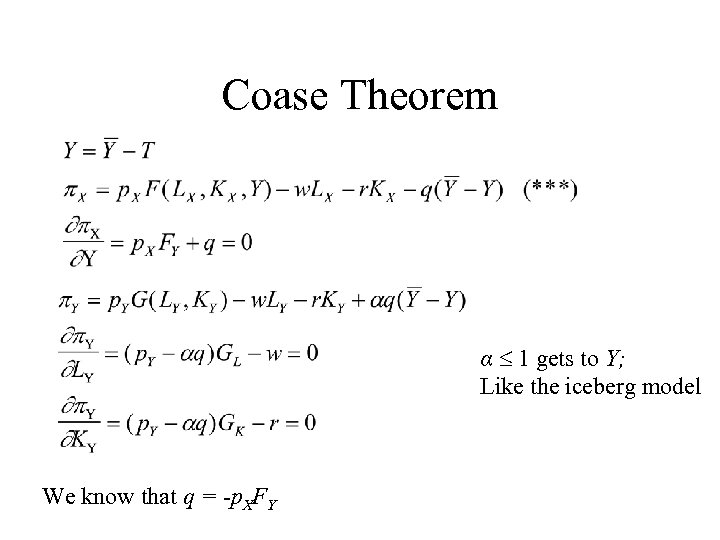Coase Theorem α 1 gets to Y; Like the iceberg model We know that q = -p. XFY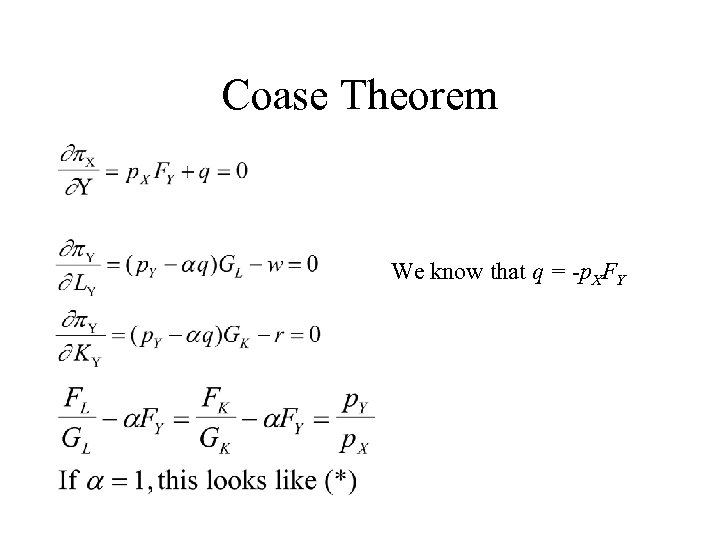Coase Theorem We know that q = -p. XFY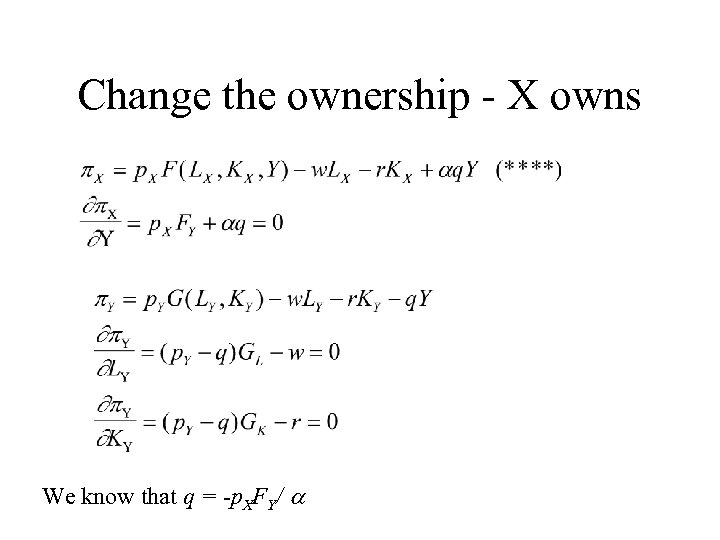Change the ownership - X owns We know that q = -p. XFY/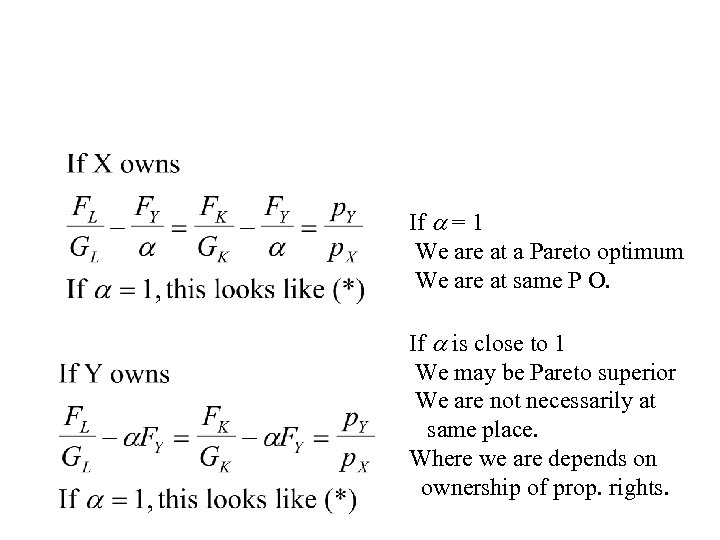If = 1 We are at a Pareto optimum We are at same P O. If is close to 1 We may be Pareto superior We are not necessarily at same place. Where we are depends on ownership of prop. rights.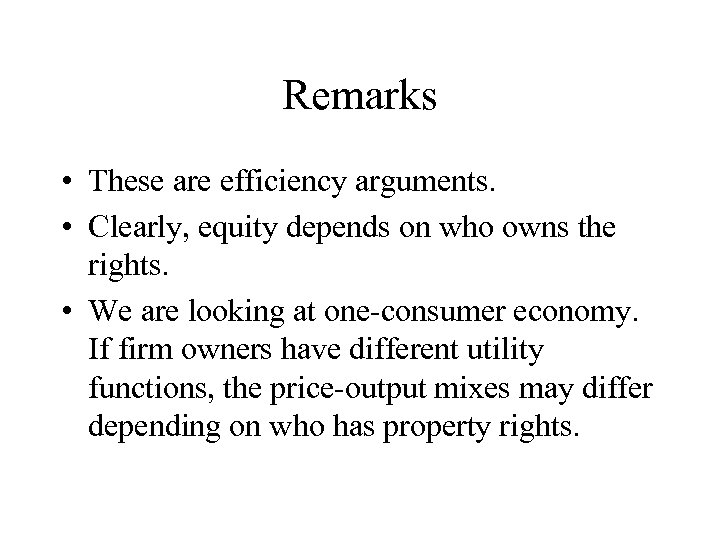Remarks • These are efficiency arguments. • Clearly, equity depends on who owns the rights. • We are looking at one-consumer economy. If firm owners have different utility functions, the price-output mixes may differ depending on who has property rights.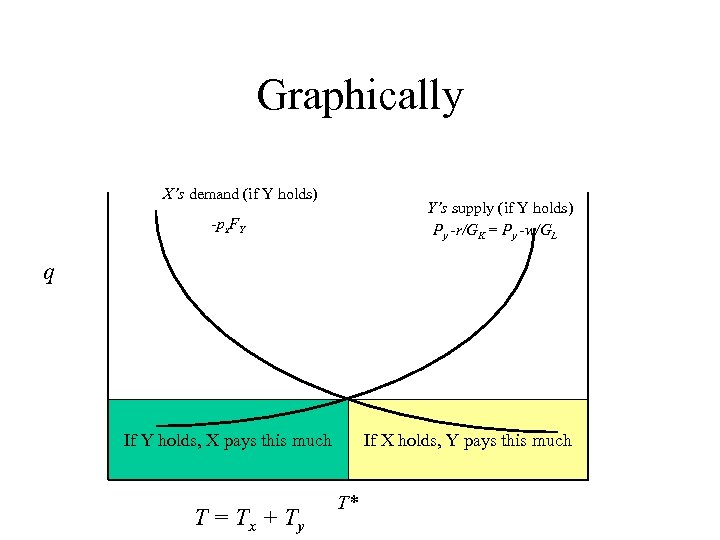Graphically X’s demand (if Y holds) Y’s supply (if Y holds) Py -r/GK = Py -w/GL -px. FY q If Y holds, X pays this much T = Tx + Ty If X holds, Y pays this much T*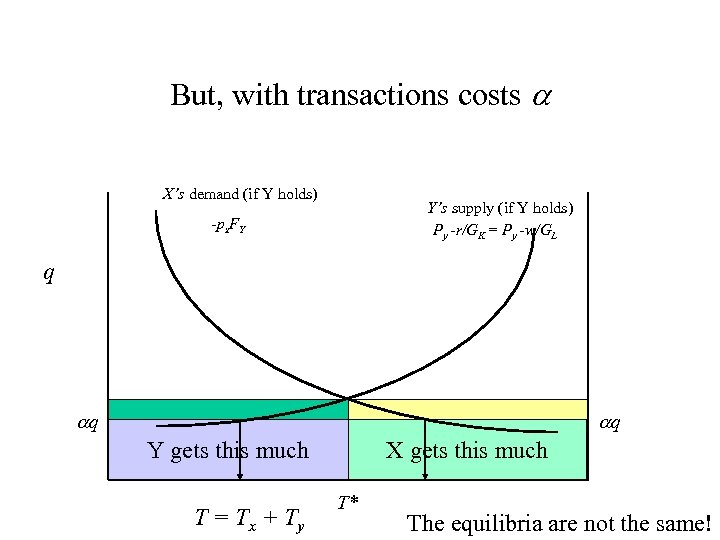But, with transactions costs X’s demand (if Y holds) Y’s supply (if Y holds) Py -r/GK = Py -w/GL -px. FY q q If Y holds, X pays this much If X holds, Y pays this much Y gets this much T = Tx + Ty q X gets this much T* The equilibria are not the same!